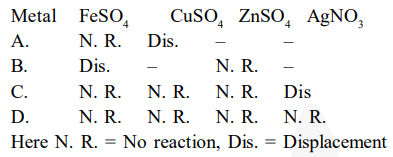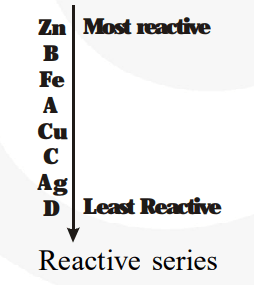# Samples of four metals A, B, C and D

Question.
Samples of four metals A, B, C and D were taken and added to the following solutions one by one. The results obtained have been tabulated as follows.Use the above table to answer the following questions about metals A, B, C and D.

(i) Which is the most reactive metal?

(ii) What would you observe if B is added to a solution of copper (II) sulphate ?

(iii) Arrange the metals A, B, C and D in the order of decreasing reactivity.

solution:
$\mathrm{A}+\mathrm{FeSO}_{4} \rightarrow$ No reaction, i.e., $\mathrm{A}$ is less reactive than iron

$\mathrm{A}+\mathrm{CuSO}_{4} \rightarrow$ Displacement, i.e., $\mathrm{A}$ is more reactive than copper

$\mathrm{B}+\mathrm{FeSO}_{4} \rightarrow$ Displacement, i.e., $\mathrm{B}$ is more reactive than iron

$\mathrm{B}+\mathrm{ZnSO}_{4} \rightarrow$ No reaction, i.e., $\mathrm{B}$ is less reactive than zinc

$\mathrm{C}+\mathrm{FeSO}_{4} \rightarrow$ No reaction, i.e., $\mathrm{C}$ is less reactive than iron

$\mathrm{C}+\mathrm{CuSO}_{4} \rightarrow$ No reaction, i.e., $\mathrm{C}$ is less reactive than copper

$\mathrm{C}+\mathrm{ZnSO}_{4} \rightarrow$ No reaction, i.e., $\mathrm{C}$ is less reactive than zinc

$\mathrm{C}+\mathrm{AgNO}_{3} \rightarrow$ Displacement, i.e., $\mathrm{C}$ is more reactive than silver

$\mathrm{D}+\mathrm{FeSO}_{4} / \mathrm{CuSO}_{4} / \mathrm{ZnSO}_{4} / \mathrm{AgNO}_{3} \rightarrow$ No reaction, i.e., $\mathrm{D}$ is less reactive than iron, copper, zinc, and silver.

From the above equations, we obtain:(i) B is the most reactive metal.

(ii) If $\mathrm{B}$ is added to a solution of copper (II) sulphate, then it would displace copper and blue colour of $\mathrm{CuSO}_{4}$ will discharge.

$\mathrm{B}+\mathrm{CuSO}_{4}-$ Displacement

(iii) The arrangement of the metals in the order of decreasing reactivity is$B>A>C>D$